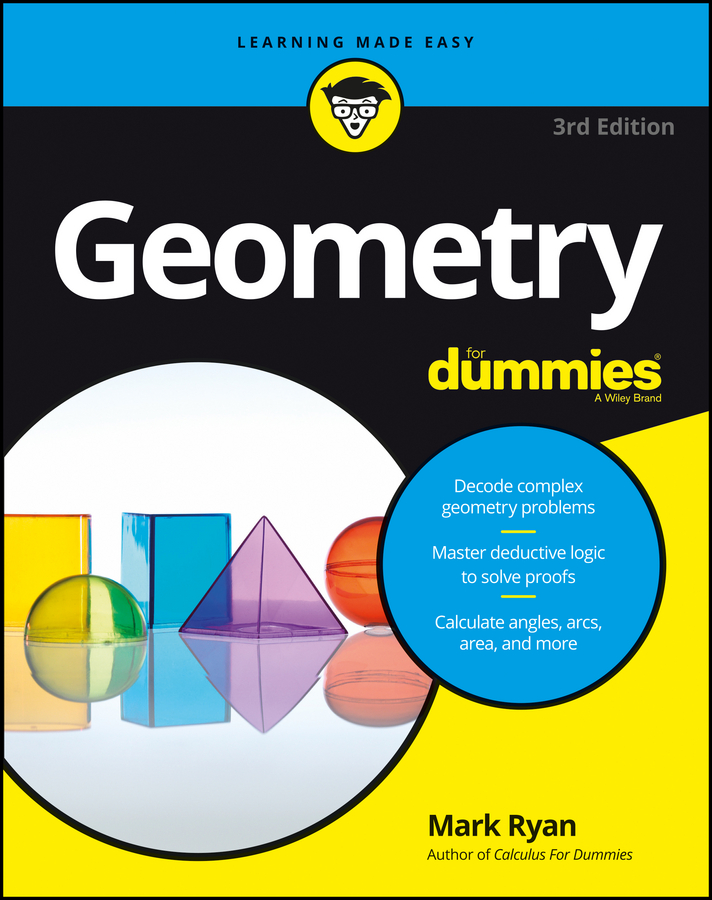##### Geometry For DummiesSay you're in the middle of a two-column geometric proof and you can't see how to get to the finish line from where you are. What do you do? One solution is to jump to the end of the proof and work backward.

Try an example:

Here's the proof diagram.

Let's say you manage to complete five lines of the proof, but you get stumped at "angle 3 is congruent to angle 4."

Where to now? Going forward from here may be a bit tricky, so work backward. You know that the final line of the proof has to be the prove statement:

Now, if you think about what the final reason has to be or what the second-to-last statement should be, it shouldn't be hard to see that you need to have two congruent angles (the two half-angles) to conclude that a larger angle is bisected.

Here's what the end of the proof looks like.

Note the if-then logic bubbles (if clauses in reasons connect to statements above; then clauses in reasons connect to statements on the same line).

Okay, so picking up where you left off on the proof: You've completed five lines of the proof, and you're up to

Now go to the end, and try working your way backward to the third-to-last statement, the fourth-to-last statement, and so on. (Working backward through a proof always involves some guesswork, but don't let that stop you.) Why might angle 7 be congruent to angle 8? Well, you probably don't have to look too hard to spot the pair of congruent vertical angles, 5 and 8, and the other pair, 6 and 7.

Okay, so you want to show that angle 7 is congruent to angle 8, and you know that angle 6 equals angle 7 and angle 5 equals angle 8. So if you were to know that angles 5 and 6 are congruent, you'd be home free.

Now that you've worked backward a number of steps, here's the argument in the forward direction: The proof could end by stating in the fourth-to-last statement that angles 5 and 6 are congruent, then in the third-to-last that angle 5 is congruent to angle 8 and angle 6 is congruent to angle 7 (because vertical angles are congruent), and then in the second-to-last that angle 7 is congruent to angle 8 by the Transitive Property (for four angles). The next figure shows how this all looks written out in the two-column format.

You can now finish by bridging the gap between statement 5

and the 4th-to-last statement

Say that congruent angles 3 and 4 are each 55 degrees. Angle 5 is complementary to angle 3, so if angle 3 were 55 degrees, angle 5 would have to be 35 degrees. Angle 6 is complementary to angle 4, so angle 6 also ends up being 35 degrees. That does it: angles 5 and 6 are congruent, and you've connected the loose ends!